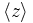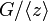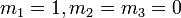# Second center not is local powering-invariant in solvable group

This article gives the statement, and possibly proof, of the fact that in a group satisfying the property solvable group, the subgroup obtained by applying a given subgroup-defining function (i.e., second center) need not satisfy a particular subgroup property (i.e., local powering-invariant subgroup)
View all such subgroup property satisfactions OR View more information on subgroup-defining functions in solvable groups

## Statement

It is possible to have a solvable group$G$ such that the second center of$G$ is not a local powering-invariant subgroup of$G$. In other words, there exists an element$g$ in the second center and a natural number$n$ such that the element$g$ has a unique$n^{th}$ root in$G$ but this$n^{th}$ root is not inside the second center.

## Proof

Define (here 1 denotes the identity element):$G := \langle x,y,z \mid y^2 = x^2z, [x,z] = [y,z] = 1 \rangle$

We can understand the structure of$G$ using the following normal series:$1 \le \langle z \rangle \le \langle x^2, z \rangle \le \langle x^2, xy, z \rangle \le \langle x,y,z \rangle$

The successive quotients are$\mathbb{Z}, \mathbb{Z}, \mathbb{Z}, \mathbb{Z}/2\mathbb{Z}$. More details below:

•$\langle z \rangle$ is the center and the quotient group$G/\langle z \rangle$ is isomorphic to the amalgamated free product$\mathbb{Z} *_{2\mathbb{Z}} \mathbb{Z}$, with the two pieces generated by the images of$x$ and$y$ and the amalgamated part being the image of$x^2$, which coincides with the image of$y^2$.
•$\langle x^2, z\rangle$ is the second center and the quotient group$G/\langle x^2,z \rangle$ is isomorphic to the free product$\mathbb{Z}/2\mathbb{Z} * \mathbb{Z}/2\mathbb{Z}$, which in turn is isomorphic to the infinite dihedral group (where the images of$x$ and$y$ are both reflections whose product gives a generator for the cyclic maximal subgroup).
•$\langle x^2, xy, z \rangle/\langle x^2, z\rangle$ is the cyclic maximal subgroup inside$G/\langle x^2,z \rangle$.

Consider now the element$g = x^2$. We have the following:

•$G$ is solvable: This is obvious from the normal series for$G$ where all the quotients are abelian.
•$g$ is in the second center, based on the description of the second center as$\langle x^2,z \rangle$.
•$g$ has a unique square root, namely$x$, in$G$: This requires some work to show rigorously, and can be demonstrated using a polycyclic presentation with the elements$x,u,z$ where$u = xy$. The idea is to compute the general expression for the square of an arbitrary element that is of the form$x^{m_1}u^{m_2}z^{m_3}$ and deduce that, for the square to equal$g$, we must have$m_1 = 1, m_2 = m_3 = 0$.
• The unique square root of$g$ is not in the second center: This follows from the explicit description of the second center and the information above.